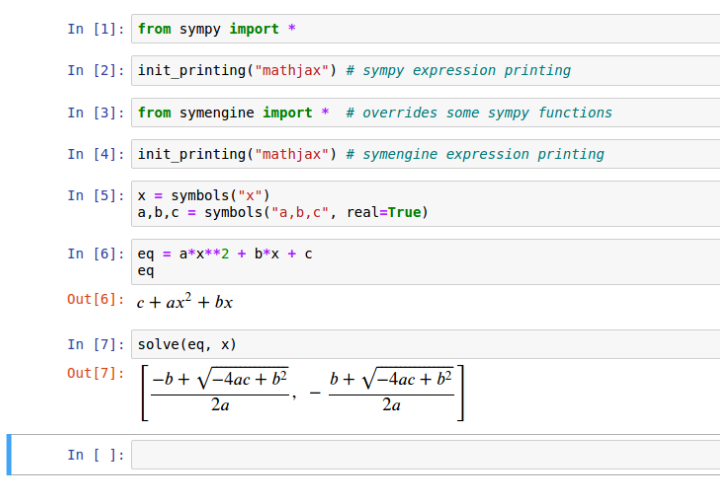# Computer Algebra With Sympy and Jupyter## Introduction

In this article, we briefly discuss the benefits and challenges of present-day computer algebra systems. Our primary motivation is how to use computer algebra systems to automate the manipulating mathematical expressions instead of having to rely on tedious and error-prone manual methods. The article presents a practical guide on how to install and work with the open-source computer algebra system, SymPy, in the Jupyter notebook. We made an example notebook available as a GitHub gist.

## Definitions

First off, we present some relevant definitions related to the topic of this article.

• Algebra is the study of mathematical symbols and the rules for manipulating these symbols. It is one of the fundamental parts of mathematics, and it is essential for conducting mathematics, science, and engineering.

• Computer algebra, a part of computational mathematics, studies algorithms for manipulating mathematical expressions and other mathematical objects. It can be though, as meta-mathematics, as it relies on mathematical methods for manipulating mathematical expressions.

• Computer algebra system is any mathematical software with the ability to manipulate mathematical expressions. General-purpose computer algebra system aims to be useful to any user working in any scientific field that requires manipulation of mathematical expressions.

In the next section, we will discuss the differences between manual and computational algebraic expression manipulation.

## Benefits of Computer Algebra Systems

Traditionally, the manipulation of mathematical expressions has required a person skilled in mathematics and lots of mental stamina. In general, handling mathematical expressions is a tedious and error-prone task for humans, and requires extensive training. In order to overcome these deficiencies, we require the aid of computer algorithms.

The emergence of computers in the 20th century has spiked an interest in algorithmic manipulation of mathematical expressions. The development of algorithms for performing algebraic manipulations has allowed a computer to perform this task. These algorithms are then bundled in computer algebra systems and made available to users.

We have listed some of the benefits of the use of computer algebra systems below.

• The manipulation of larger expressions is feasible.
• The manipulation of expressions can be automated, which is especially useful when the same operations are performed multiple times for different inputs.
• There is a decreased risk of error when manipulating expressions computationally.
• Results are much easier to share. Also, online sharing is possible.
• The computer is better at representing mathematical expressions. There is no disambiguation between symbols.

However, computer algebra systems are not without their challenges.

• The learning curve of computer algebra systems is often quite steep. Initially, even simple operations can require more work compared if done by hand.
• Computer algebra systems may not have implemented all required capabilities, which may require manual intervention.

In conclusion, we will still need to rely on a hybrid approach where we combine manual and computational work. As technology improves, we can perform an increasing quantity of our algebraic manipulations using computers. In the next section, we discuss open-source computer algebra systems and how to use them.

## Using Jupyter and SymPy

This part focused on open-source software for computer algebra. We will discuss how to use the Jupyter notebook and Python programming language with the SymPy library. Additionally, we install the SymEngine library for improved performance of symbolic operations. Each of the software can be installed using the Conda package manager.

1. Install the Conda package manager. We recommend installing the Miniconda distribution with the latest Python interpreter.

2. Now Jupyter and SymPy can be installed using Conda using the command

conda install jupyter sympy

3. Additionally, we may choose to install SymEngine and its Python wrappers

conda install python-symengine symengine -c symengine -c conda-forge

4. Jupyter notebook is started using the command

jupyter notebook

5. Create a new Jupyter notebook and select the Python kernel.

6. Include the following lines of code at the beginning of the Jupyter notebook. First-line imports all the functions from SymPy, making them available to the global variables. The second line enables math rendering in the browser, similarly to LaTeX via MathJax.

from sympy import *
init_printing("mathjax")


Now we are ready to start working with SymPy. Take a look at the SymPy examples Jupyter notebook. It contains a showcase of the essential SymPy features.

SymPy and SymEngine is also available for Julia programming language through the wrappers Sympy.jl and SymEngine.jl. Julia kernel can be added to Jupyter using IJulia.jl package.

## Contribute

If you enjoyed or found benefit from this article, it would help me share it with other people who might be interested. If you have feedback, questions, or ideas related to the article, you can write to my GitHub Discussions forum.

***

For more content, you can follow my YouTube channel and join my newsletter. Since creating content and open-source libraries take time and effort, consider supporting the effort by subscribing or giving a one-time donation.##### Jaan Tollander de Balsch
###### Computational Scientist

Jaan Tollander de Balsch is a computer scientist with a background in applied mathematics.